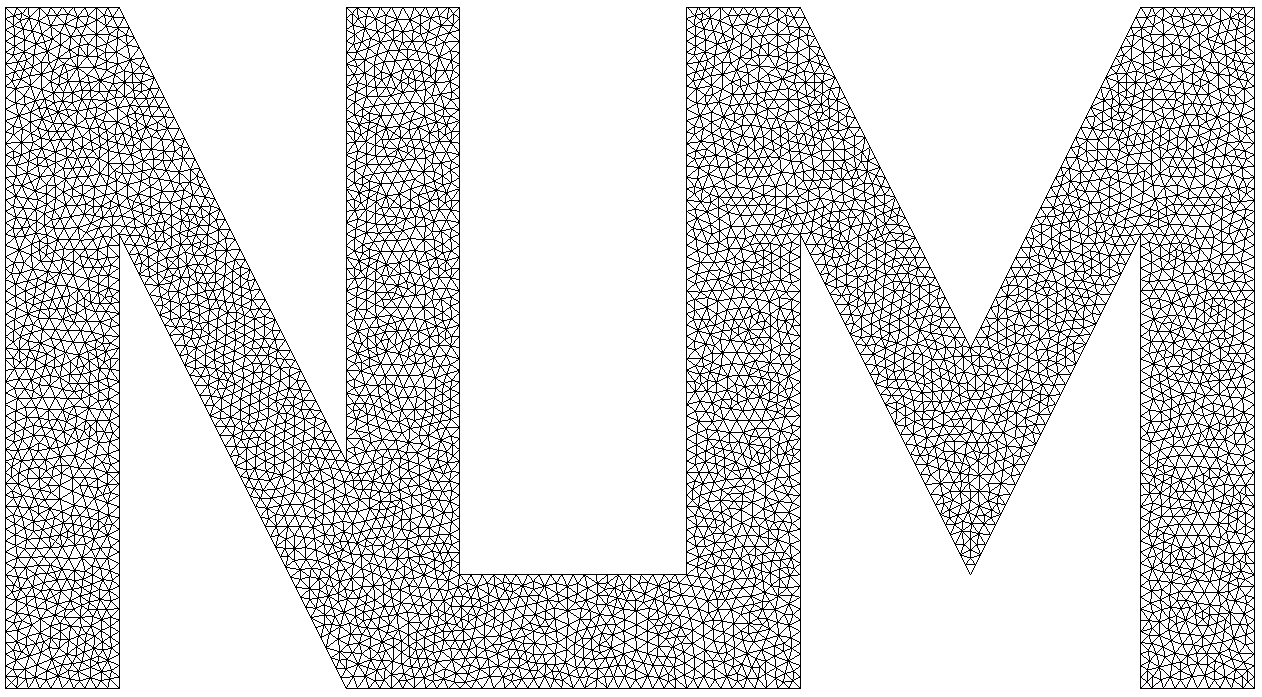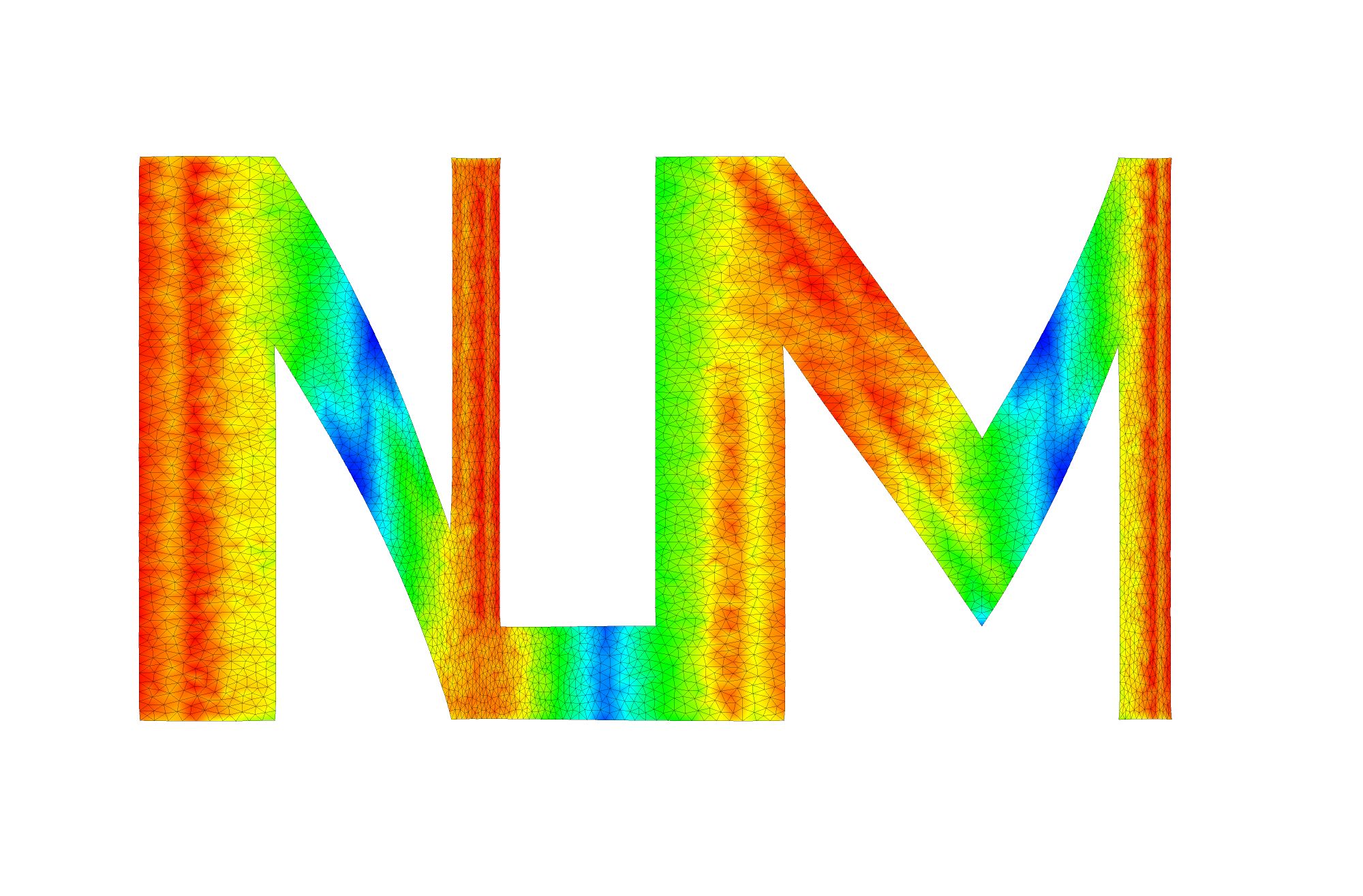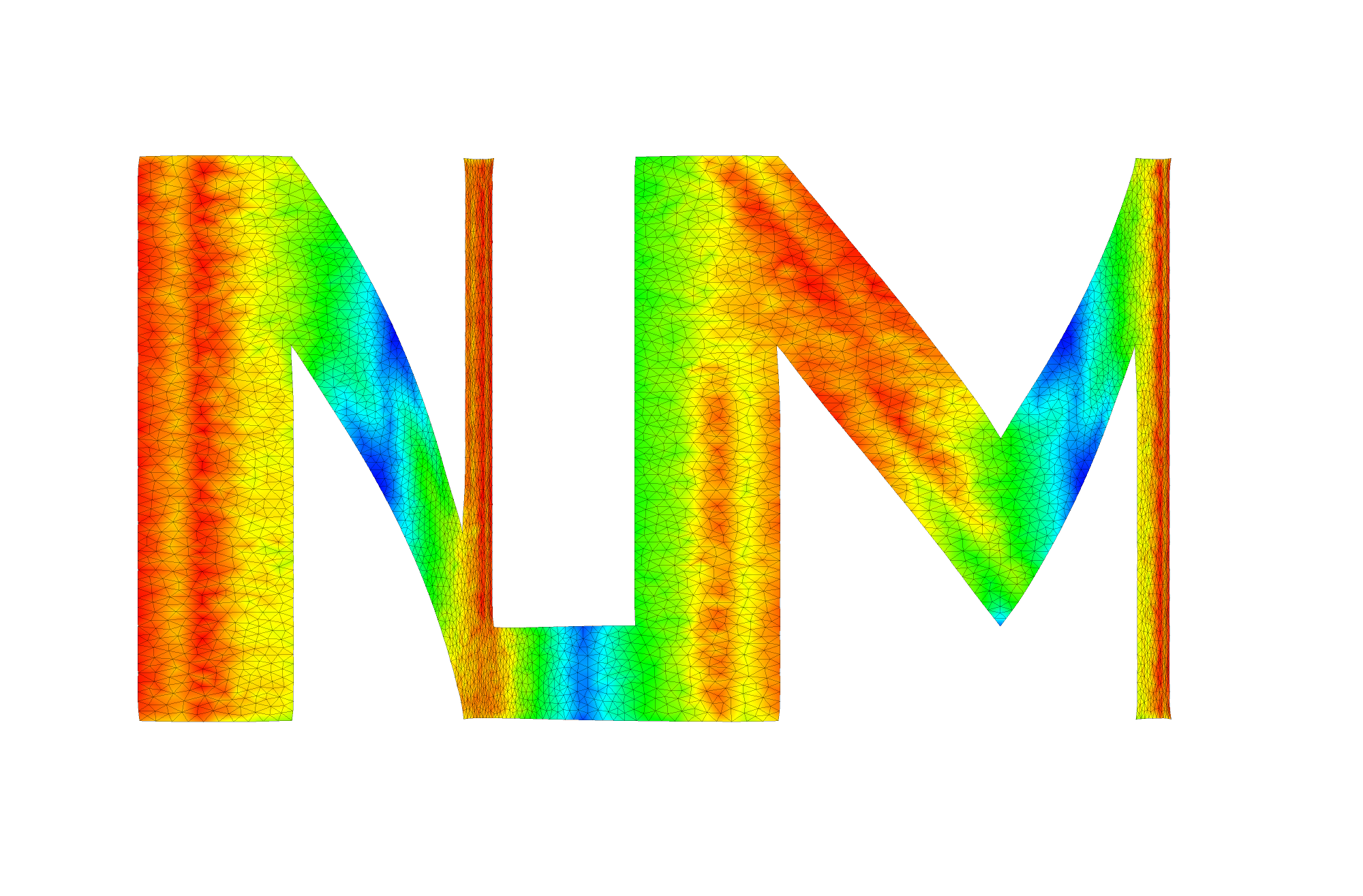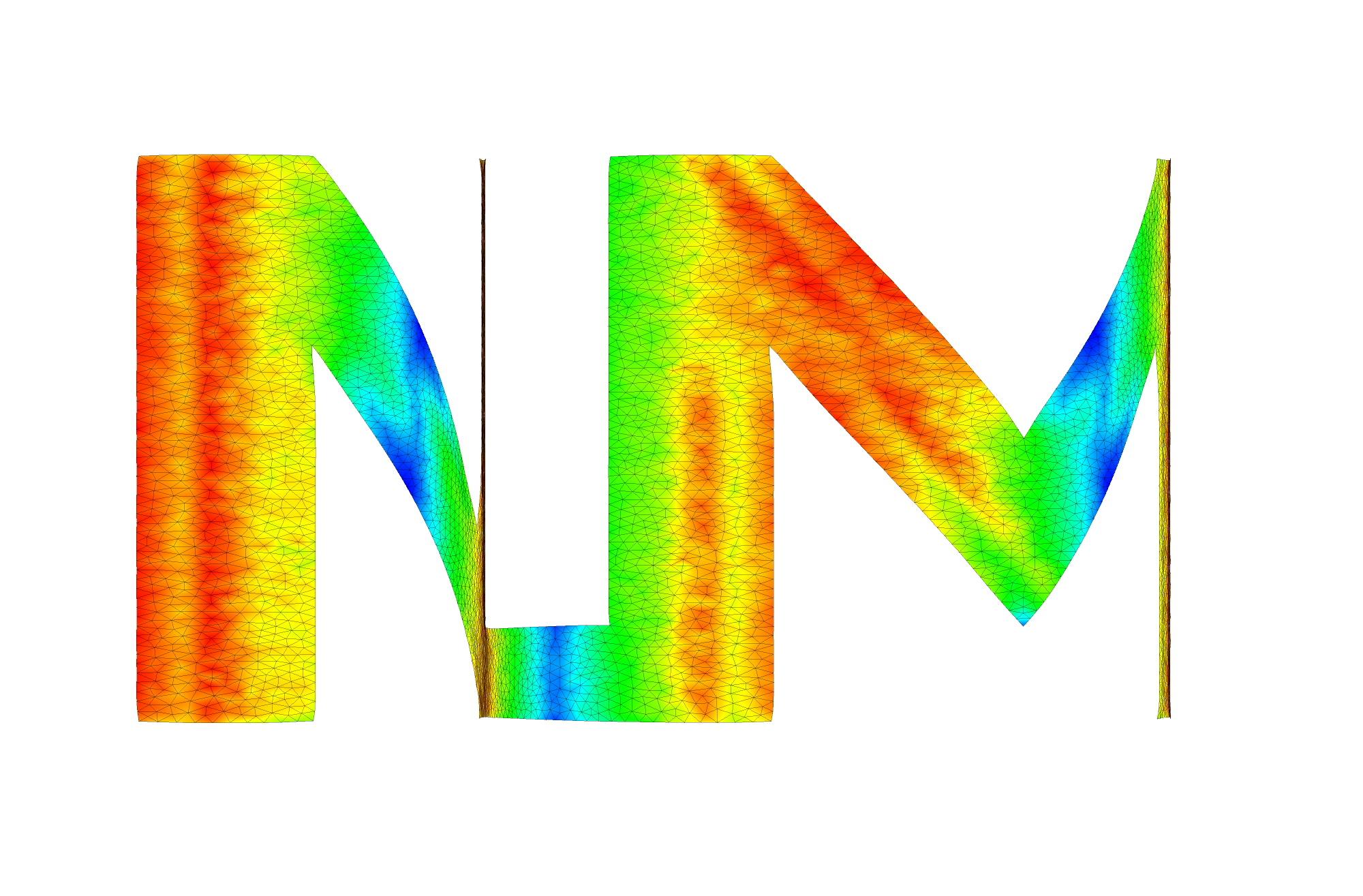• We consider a Peridynamic simulation of Soft material using RNP Peridynamic model (Regularized Nonlinear Peridynamic) developed and studied in Lipton 2016 and Jha and Lipton 2018. RNP model is implemented in class RNPBond.

• Units are SI units, e.g. length is in meter, mass is in kg, force is in N, and time is in second.

• Elastic properties of material are as follows:

• Shear modulus G = 35.2E+03 Pa
• Poisson ratio $\nu$ = 0.25
• Density $\rho$ = 1011.204 kg/$m^3$
• Fracture properties of material are as follows:

• Critical energy release rate $G_c$ = 9.998E+02 J/$m^2$
• For above elastic properties and fracture properties, we can compute the parameters in Peridynamic material model. This has been described in detail in RNPBond::computeParameters.

• We consider a triangular mesh for “NLM” logo. It is contained in the box [0, 2.75 m] x [0, 1.5 m].

• Horizon is $\epsilon$ = 0.1 m and mesh size is h = 0.025 m.

• Time domain

• Final time T = 0.01
• Time steps N = 50000
• Output frequency of simulation data: N/100
• Boundary condition

• We apply body force along horizontal axis on whole material domain.

• Force is given by $f_x(x,y,t) = f t sin(n\pi x/L_x)$ where n = 3 and f = 1.5E+09.

• We specify Displacement, Velocity, Force and Damage_Z as output candidates.

### Mesh

We obtain mesh using Gmsh library with mesh size h = 0.025.### Results

We show damage plot at time t = 0.008, 0.009, 0.01 seconds.Video of simulation: